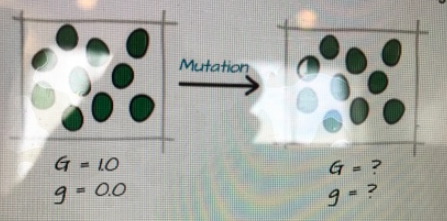# Problem: Calculate the allele frequencies in the iguana population following the mutation event.A. 0.9 GG, 0.1 Gg B. 9 GG, 1 Gg, 0 ggC. 0.95 G, 0.05 g D. 0.9 G, 0.1 g E. 1.0 G, 0.0 g

###### FREE Expert Solution

Hardy-Weinberg Equation:

${\mathbf{p}}^{\mathbf{2}}\mathbf{+}\mathbf{2}\mathbf{pq}\mathbf{+}{\mathbf{q}}^{\mathbf{2}}\mathbf{=}\mathbf{1}$

where: p2 = frequency of homozygous dominant (NAA/N)

2pq = frequency of heterozygous (NAa/N)

q2 = frequency of homozygous recessive (Naa/N)

= frequency of dominant allele

q = frequency of recessive allele

Given: after the mutation event

NAA = 9          NAa = 1          N = 10###### Problem Details

Calculate the allele frequencies in the iguana population following the mutation event.A. 0.9 GG, 0.1 Gg

B. 9 GG, 1 Gg, 0 gg

C. 0.95 G, 0.05 g

D. 0.9 G, 0.1 g

E. 1.0 G, 0.0 g